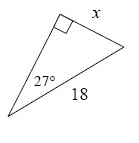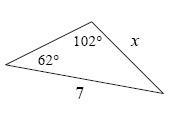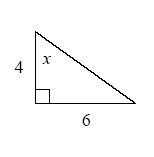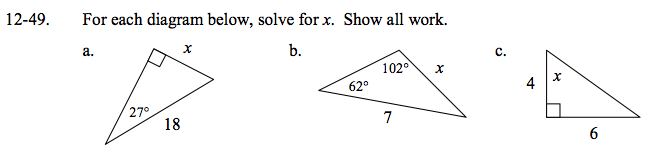### Home > GC > Chapter 12 > Lesson 12.1.5 > Problem12-49

12-49.
1. For each diagram below, solve for x. Show all work. Homework Help ✎

1.2.3.What trigonometric ratio can you use to find the value of x?

x = 8.17

Use the Law of Sines to find x.

$\frac{ \text{sin}(102^{\circ}) }{7 }= \frac{ \text{sin}(62^{\circ}) }{x }$

x = 6.32

What trigonometric ratio can you use to find x?